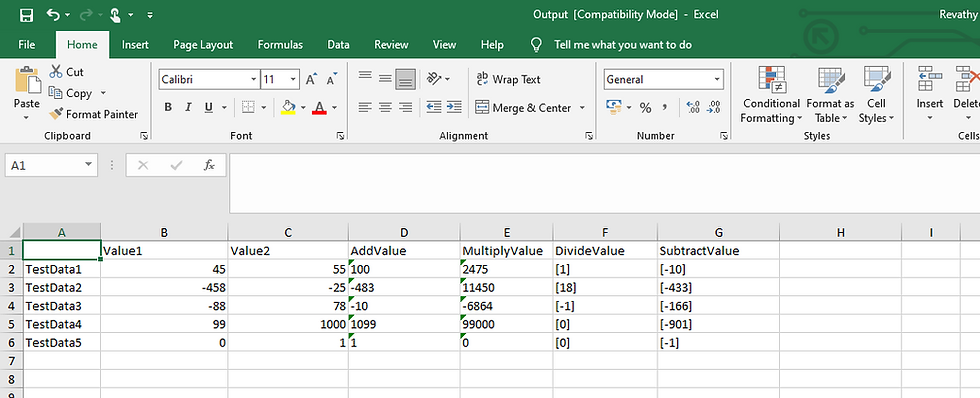top of page
Search

# Automating Test data through Excel file in SOAP UI using groovy script

Updated: Dec 30, 2020

Please refer previous tutorials for creating properties and accessing them in test suites and test cases.

Let us consider our calculator example,

We have four request XMLs to Add, Divide, Multiply and Subtract. Let's create a new test suite with all four requests to access values from excel file.

Step 1: create Test Suite and add required test requests. To learn how to create test suite and test steps refer Creating your first SOAP UI test.Step 2:

Create a testdata excel file with data needs to be passed to the request XMLs.

Note: If you find any error while executing the code, save the excel file as ".xls" instead of .xlsxStep 3:

Create a properties test step to store values from excel sheet. To learn how to create properties refer Properties in Soap UI.

Create two properties, "ValueOne" and "ValueTwo" in properties test step.Step 4:

Unzip the file, and copy JXL.jar to your SOAPUI installation folder.

i.e. SOAPUI-> bin -> extStep 5:

Create a groovy script step by doing right click -> Add step -> Groovy Script. And add following groovy code to read data from excel data.```//import required jars

import com.eviware.soapui.support.XmlHolder
import jxl.*
import jxl.write.*

//define the test case where TestCaseOne is the name of your test case
def myTestCase = context.TestCaseOne

//define Excel variables
Workbook workbook1 = Workbook.getWorkbook(new File("C:\\revprojects\\TestData.xls"))
def outputDataFileName = "C:\\revprojects\\Output.xls"
Workbook existingWorkbook = Workbook.getWorkbook(new File("C:\\revprojects\\TestData.xls"));
WritableWorkbook workbookCopy = Workbook.createWorkbook(new File(outputDataFileName), existingWorkbook);
WritableSheet sheetToEdit = workbookCopy.getSheet(0);

//Index 0 will read the first sheet from the workbook, you can also specify the sheet name with "Sheet1"
Sheet sheet1 = workbook1.getSheet(0)
WritableCell Mulcell;
WritableCell Subcell;
WritableCell Divcell;

//To get the number of rows
size= sheet1.getRows().toInteger()

//Iterate no of rows to get corresponding value
for(int i = 1;i<size;i++) {
Cell v1 = sheet1.getCell(1,i)  			//points to value1 and value2 from excel
Cell v2 = sheet1.getCell(2,i)

//set the values to properties test step and run the requests via groovy
testRunner.testCase.getTestStepByName("Properties").setPropertyValue("ValueOne",v1.getContents())
testRunner.testCase.getTestStepByName("Properties").setPropertyValue("ValueTwo", v2.getContents())
testRunner.testCase.getTestStepByName("MultiplyStep").run(testRunner, context)
testRunner.testCase.getTestStepByName("DivideStep").run(testRunner, context)
testRunner.testCase.getTestStepByName("SubtractStep").run(testRunner, context)

//get the values from response xml
def Multiplyholder = context.expand('\${MultiplyStep#response}')
def Multiplyresponse = new XmlHolder(Multiplyholder)
def multiplyvalue = Multiplyresponse.getNodeValue( "//*:MultiplyResult" )
log.info multiplyvalue.toString()
def Divideholder = context.expand('\${DivideStep#response}')
def Divideresponse = new XmlHolder(Divideholder)
def Dividevalue = Divideresponse.getNodeValues( "//*:DivideResult" )
log.info Dividevalue.toString()
def Subholder = context.expand('\${SubtractStep#response}')
def Subresponse = new XmlHolder(Subholder)
def subtractvalue = Subresponse.getNodeValues( "//*:SubtractResult" )
log.info subtractvalue.toString()

//update the values in excel
Label MulL = new Label(4, i,multiplyvalue);
Mulcell = (WritableCell) MulL;
Label DivL = new Label(5, i,Dividevalue.toString());
Divcell = (WritableCell) DivL;
Label SubL = new Label(6, i,subtractvalue.toString());
Subcell = (WritableCell) SubL;

}
workbookCopy.write();
workbook1.close();
workbookCopy.close();

```

This overall function of this code is,

1. get the data from input excel from specified path

2. inside for loop, iterate each row from excel and assign the values to properties in properties test step.

3. run each test request within loop

4. extract the output result from response xml

5. write the output values to new output excel file.

Step 6:

Move "groovy script" test step and "properties" test step to beginning and disable all other requests from the test steps. Those requests have already been called inside loop in groovy script step.Step 7:

Run the groovy script and the log file displays all the output values extracted from repsonse.Step 8:

Now the test case is passed and output file generated in specified path with expected values.